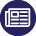#POST

OCT
5
2021

## Closed form Fibonacci

ORIGINALLY POSTED TO: https://blog.simontimms.com/2021/10/05/fibonacci

A favorite programming test question is the Fibonacci sequence. This is defined as either `1 1 2 3 5...` or `0 1 1 2 3 5...` depending on what you feel fib of 0 is. In either case fibonacci is the sum of the two previous terms. So fib(10) = fib(9) + fib(8). The reason this is a programming test favorite is because it forces people to think about recursion and even memoization for performance.

There is however a shortcut for this and that is to use the closed form which uses the golden ratio. We have two interesting values called Phi and phi with the former being the golden ratio and the latter being a value observed in nature for leaf dispersions.

We can use this to create Binet's formula (Jacques Philippe Marie Binet was a French mathematician born in 1786, although this formula is named for him it was really discovered by fellow French mathematician Abraham do Moivre a century earlier)

In code we can do the following to generate all the fib numbers up to n

The advantage here is that the closed form is constant time, constant memory and uses only about 3 64-bit values (fewer if you calculate phi and Phi as you go). It's certainly a lot of fun to break out in a programming test.